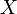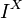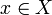# 10-327/Homework Assignment 8

## Contents

### Change in Plans

Time to rest, a bit. For the lack of fun questions, I've decided to postpone this assignment a bit, and to cancel assignment number 9. So this assignment will only be written on Monday November 29, and will be due on the following Monday, December 6.

### Doing

Solve and submit the following problems from Munkres' book:

• Problems 2, 5a on pages 223-224.
• Problems 1, 4, 5 on page 270 (the relevant material will be covered on Thursday December 2).
• Problem 1 on page 280 (same comment).

In addition, solve and submit the following problem:

Problem IX. Show that every metric space$X$ can be embedded in the cube$I^X$.

Hint. Given a point$x\in X$, what real-valued function on$X$ immediately comes to mind?

### Due date

This assignment is due at the end of class on Wednesday, December 8, 2010.

 Dror's notes above / Student's notes below

### Discussion

• Then how do we count the homework in the final grade? I remember the old rule is to select the best 7 assignments from the total of 9. - Jolin

Question. For question 4. It is to show the intersection is nonempty by creating a Cauchy sequence from these A_n's. But I can't think of a way to prove the other direction. Are we supposed to create a nested sequence of closed sets for every Cauchy sequence? Then how do we ensure closeness? -Kai

Take the closure of each set, as your set. -Jdw Yea thanks Jason. It works because the diam of the closure of a set is the same as the set in the metric topology. -Kai